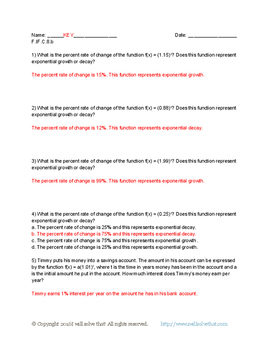# High School Math: Functions F.IF.C.8.b Free Worksheet!Subject
Resource Type
File Type

PDF

(59 KB|6 pages)
Product Rating
4.0
(3 Ratings)
Standards
• Product Description
• StandardsNEW
This free worksheet contains 10 problems aligning with Common Core standard F.IF.C.8.b. Students will determine whether functions represent exponential growth or decay and the percent rate of change. Students will then answer word problems containing exponential functions. Answer key included. 6 page PDF. This worksheet can be used as an in class assignment or as a homework assignment.
Use the properties of exponents to interpret expressions for exponential functions. For example, identify percent rate of change in functions such as y = (1.02) to the 𝘵 power, 𝘺 = (0.97) to the 𝘵 power, 𝘺 = (1.01) to the 12𝘵 power, 𝘺 = (1.2) to the 𝘵/10 power, and classify them as representing exponential growth or decay.
Total Pages
6 pages
Included
Teaching Duration
N/A
Report this Resource to TpT
Reported resources will be reviewed by our team. Report this resource to let us know if this resource violates TpT’s content guidelines.